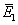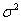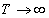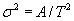NONLINEAR PHENOMENA IN COMPLEX SYSTEMS
An Interdisciplinary Journal

2006, Vol.9, No.2, pp.141-149

Testing Adiabatic Invariance in Separatrix Crossing.
Marko Robnik and Thomas A. Wood

We study the crossing of a separatrix in a time dependent 1D symmetric quartic double well potential and the time evolution of a canonical ensemble of initial conditions (a uniform distribution with respect to the angle variable on the initial contour of constant energy E0). We calculate the distribution P(E1) of the final energies E1 after the time T of the adiabatic process. In particular we calculate the mean final energyand the varianceof P(E1). We show that in the adiabatic limit when the process of the varying potential is infinitely slow (meaning) the adiabatic invariance (constant classical action integral) is fully preserved, and the final energy is in perfect agreement with the theoretical prediction. In the limitthe variance of the energy decays to zero oscillating but in the mean inversely quadratically, namely, and the constant A agrees very well with the theoretical A due to the separatrix crossing, implying that this is the dominant contribution in this adiabatic limit. The theoretical calculation of the final energy distribution P(E1) and of all its moments we leave as an important open theoretical problem, although qualitatively we understand its structure.
Key words: classical dynamics, Hamilton systems, adiabatic invariants, separatrix crossing

Full text:  Acrobat PDF  (2824KB)

Copyright © Nonlinear Phenomena in Complex Systems. Last updated: July 11, 2006